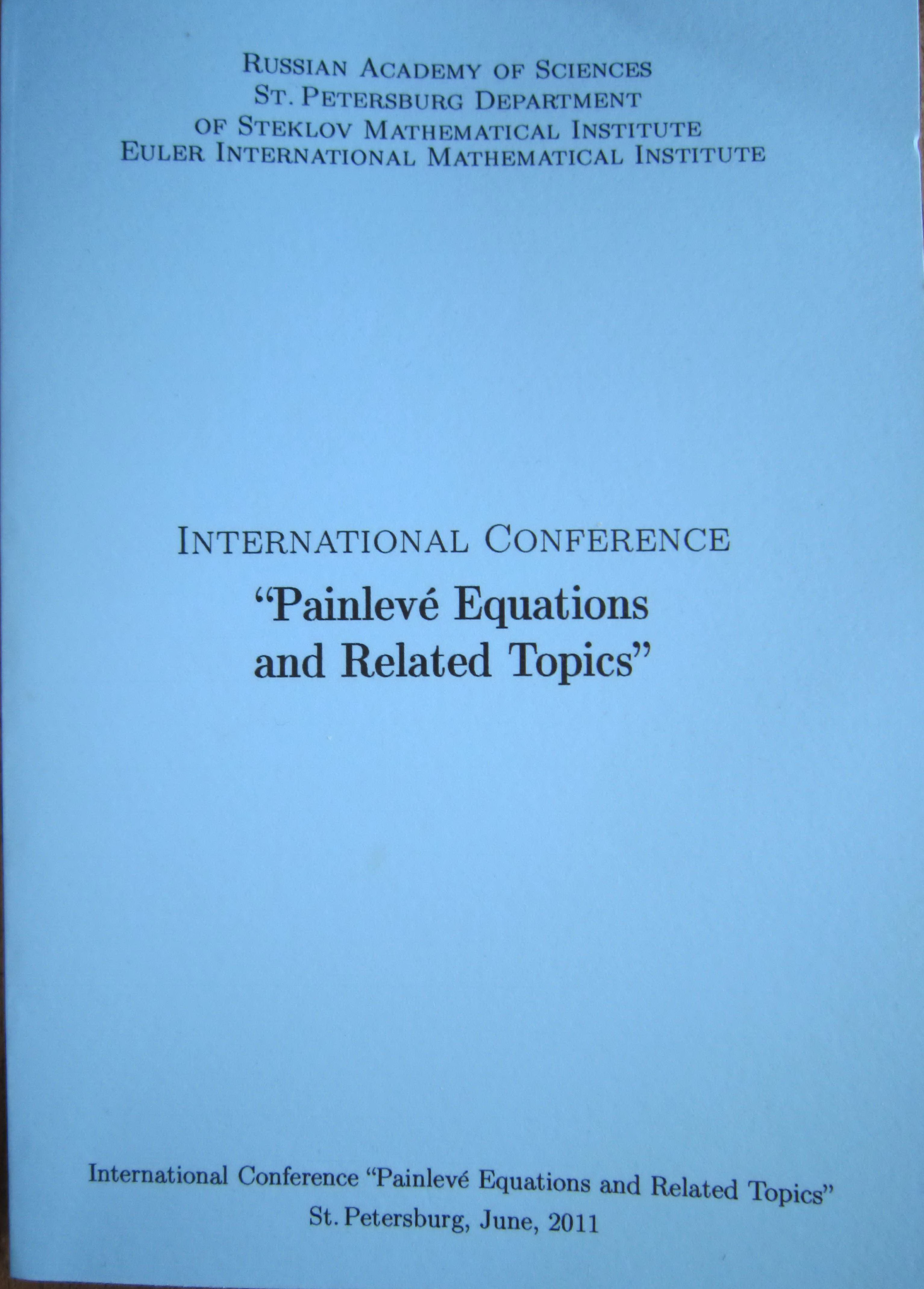• A
• A
• A
• ABC
• ABC
• ABC
• А
• А
• А
• А
• А
Regular version of the site

Asymptotic expansions of solutions to the fifth Painleve equation

P. 126-131.

By means of Power Geometry we obtained all asymptotic expansions of solutions to the equation P5 of the following five types: power, power-logarithmic, complicated, exotic and half-exotic for all values of 4 complex parameters of the equation. They form 16 and 30 families in the neighbourhood of singular points z = infty and z = 0 correspondingly. There exist 10 families in the neighbourhood of nonsingular point. Over 20 families are new.

In bookSt. Petersburg: The Euler International Mathematical Institute, 2011.